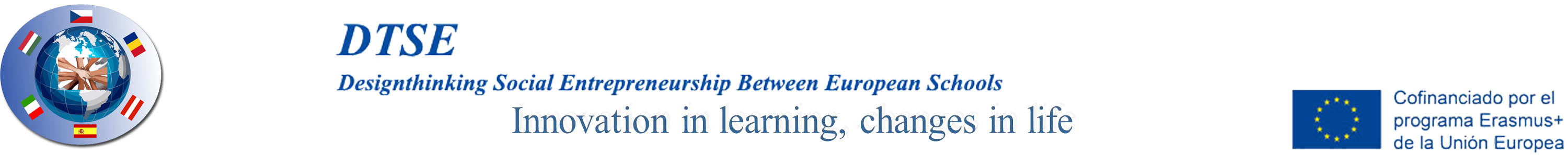3.2 Dynamic methodsDynamic methods are more complex but more precise than static methods

These methods keep in mind the value of money at the time the flow occurs. For this reason, these methods are more realistic.

Two monetary quantities that are obtained at different times have a different value, and money can be capitalized at a certain interest rate and the purchasing and the purchasing power of money changes due to inflation.

The capital we obtained (Co) after n periods of capitalization at a determined interest rate per period (i) will be equal to Cn.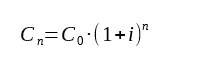If we know Cn, and we want to know the quantity before n periods of time, we apply: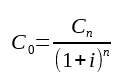Net present value

This method consists in updating all the cash flows and obtaining the current value of the capital. The quantities should be added or subtracted when they are monetary inputs or outputs caused by the investment.

Considering (i) as the interest rate: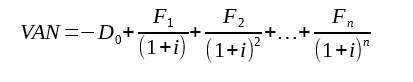If the result is negative, it means that the outputs are larger than the inputs. If this happens the investment will not be chosen

If the result is positive, it means that the outputs are larger than the inputs. In this case the investment will be developed

If the result equals 0, the outputs will be the same as the inputs, meaning that carry out the investment is indifferent.

The criteria to choose an investment according to this method, is to choose the one whose result is the highest, as long as it is positive.

Internal rate of return:

This method, represented with the letter r, makes the value of the net present value equal 0.

We calculate it: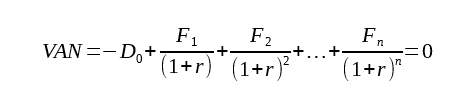The value of r gives us a measure of the profitability of the investment. To choose an investment according to this criterion we will compare the value of r to the current market interest rate (I).

If r is higher than I, the investment should be made because the profitability obtained will be higher than the one of the market. The net present value will be positive and the company will obtain a profit out the investment.

If r is lower than I, the investment should not be made because the profitability that could be obtained will be lower than to the one of the market, in this case it would more convenient to keep the money in the bank.

If r=I, carrying out the inversion is indifferent because the results obtained will only be enogh to recover the money used for the investment.

Out of all the projects of investment in which r is higher than I, we will choose the one with the highest r.